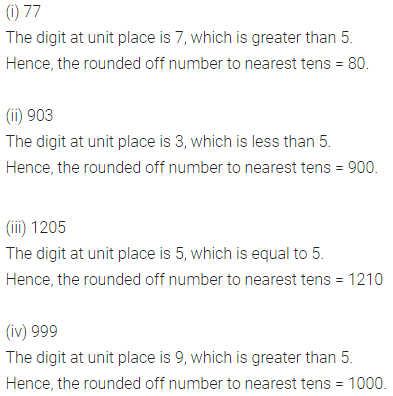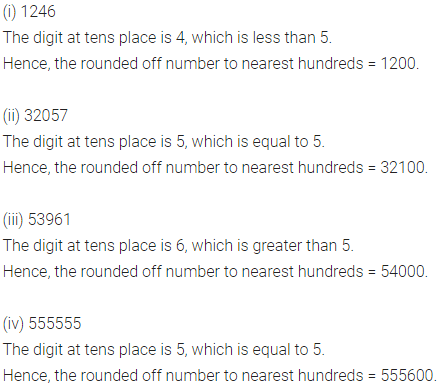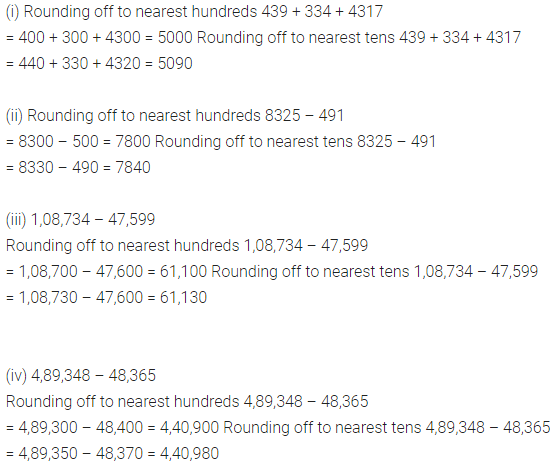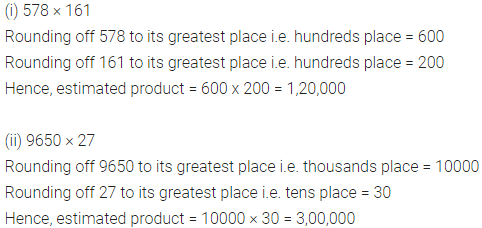# ML Aggarwal Class 6 Solutions for ICSE Maths Chapter 1 Knowing Our Numbers Ex 1.4

## ML Aggarwal Class 6 Solutions for ICSE Maths Chapter 1 Knowing Our Numbers Ex 1.4

Question 1.
Round off each of the following numbers to their nearest tens:
(i) 77
(ii) 903
(iii) 70 1205
(iv) 999
Solution:Question 2.
Estimate each of the following numbers to their nearest hundreds:
(i) 1246
(ii) 32057
(iii) 53961
(iv) 555555
Solution:Question 3.
Estimate each of the following numbers to their nearest thousands:
(i) 5706
(ii) 378
(iii) 47,599
(iv) 1,09,736
Solution:Question 4.
Give a rough estimate (by rounding off to nearest hundreds) and also a closer estimate (by rounding off to nearest tens):
(i) 439 + 334 + 4317
(ii) 8325 – 491
(iii) 1,08,734-47,599
(iv) 4,89,348 – 48,365
Solution:Question 5.
Estimate each of the following by rounding off each number nearest to its greatest place:
(i) 730 + 998
(ii) 5,290 + 17,986
(iii) 796-314
(iv) 28,292 – 21,496
Solution:Question 6.
Estimate the following products by rounding off each of its factors nearest to its greatest place:
(i) 578 × 161
(ii) 9650 × 27
Solution:Question 7.
Estimate the following products by rounding off each of its factors nearest to its hundreds place:
(i) 5281 × 3491
(ii) 1387 × 888
Solution: